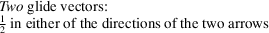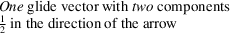International
Tables for
Crystallography
Volume A
Space-group symmetry
Edited by M. I. Aroyo

International Tables for Crystallography (2016). Vol. A, ch. 2.1, p. 146

## Table 2.1.2.3

Th. Hahna and M. I. Aroyoc
 Table 2.1.2.3| top | pdf | Graphical symbols of symmetry planes parallel to the plane of projection
DescriptionGraphical symbolGlide vector(s) of the defining operation(s) of the glide plane (in units of the shortest lattice translation vectors parallel to the projection plane)Symmetry element represented by the graphical symbol
Reflection plane, mirror planeNone m
Axial' glide planein the direction of the arrow a, b or c
Double' glide planee
Diagonal' glide planen
Diamond' glide plane§ (pair of planes)in the direction of the arrow; the glide vector is always half of a centring vector, i.e. one quarter of a diagonal of the conventional face-centred cell d
The symbols are given at the upper left corner of the space-group diagrams. A fraction h attached to a symbol indicates two symmetry planes with heights' h andabove the plane of projection; e.g.stands forand. No fraction meansand(cf. Section 2.1.3.6).
The graphical symbols of the e'-glide planes are applied to the diagrams of seven orthorhombic A-, C- and F-centred space groups, five tetragonal I-centred space groups, and five cubic F- and I-centred space groups.
§Glide planes d occur only in orthorhombic F space groups, in tetragonal I space groups, and in cubic I and F space groups. They always occur in pairs with alternating glide vectors, for instanceand. The second power of a glide reflection d is a centring vector.